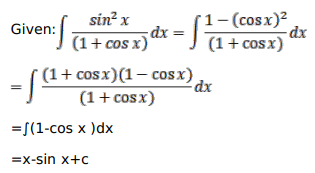# Mark against the correct answer in each of the following:

Question:

Mark $(\sqrt{ })$ against the correct answer in each of the following:

$\int \frac{\sin ^{2} x}{(1+\cos x)} d x=?$

A. $x+\sin x+C$

B. $x-\sin x+C$

C. $\sin x-x+C$

D. $-\sin x-x+C$

Solution: